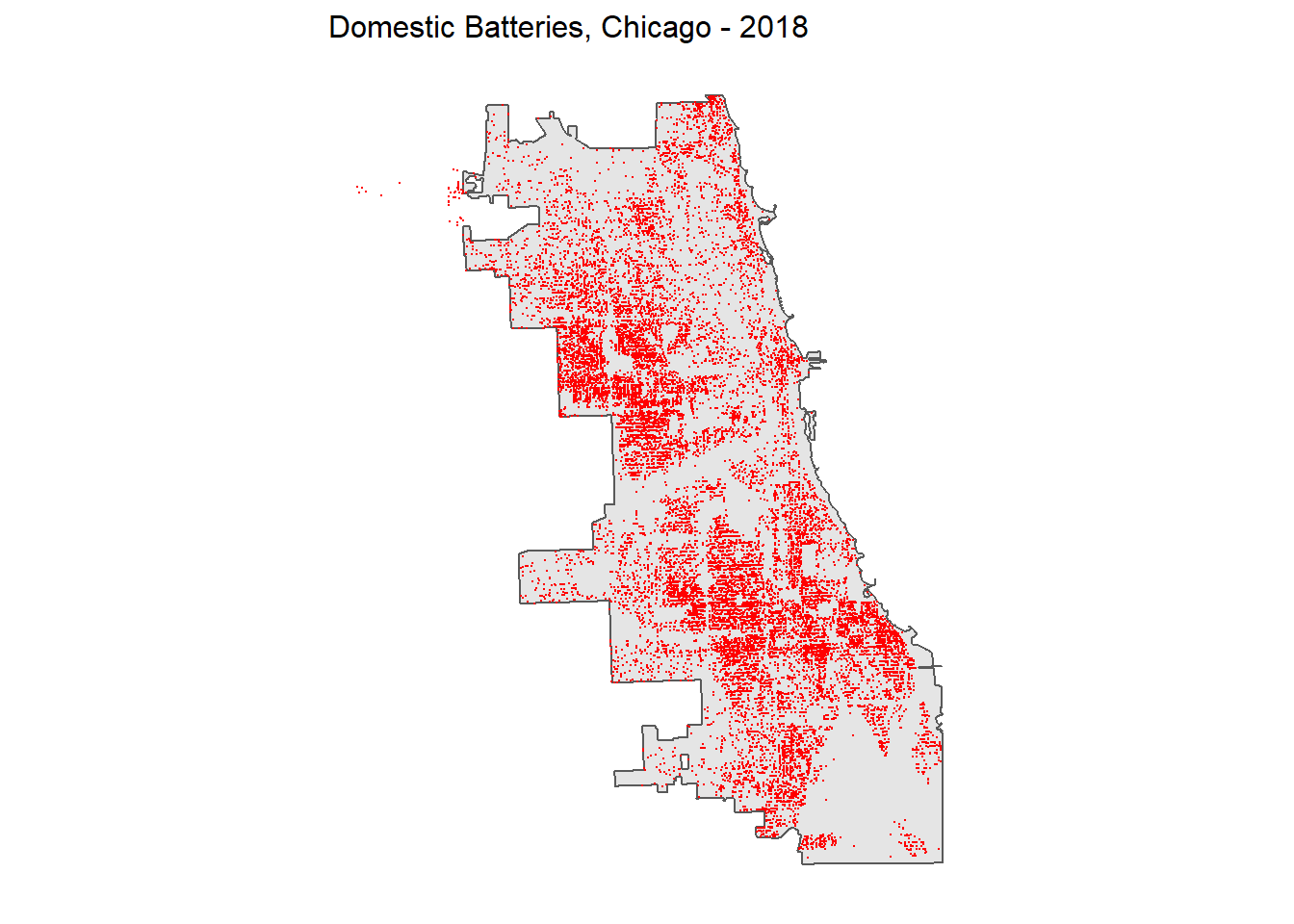In this analysis, the risk of domestic batteries in the city of Chicago is predicted using a machine learning model. Such a model could potentially increase the policing efficiency by directing officers where to focus for the patrols, but it might also suffer from the selection bias originated from different demographics. The accuracy and generalizability will be evaluated and a recommendation of whether the proposed model should be put into production will be delivered in the end.

## 1. setup

``````library(tidyverse)
library(sf)
library(QuantPsyc)
library(RSocrata)
library(viridis)
library(caret)
library(spatstat)
library(spdep)
library(FNN)
library(grid)
library(gridExtra)
library(knitr)
library(kableExtra)
library(tidycensus)

mapTheme <- function(base_size = 10) {
theme(
text = element_text(color = "black"),
plot.title = element_text(size = 12,colour = "black"),
plot.subtitle=element_text(face="italic"),
plot.caption=element_text(hjust=0),
axis.ticks = element_blank(),
panel.background = element_blank(),
axis.title = element_blank(),
strip.background = element_rect(fill = "white", color = "white"),
strip.text.x = element_text(size = 8),
axis.text = element_blank(),
axis.title.x = element_blank(),
axis.title.y = element_blank(),
panel.grid.minor = element_blank(),
#panel.border = element_rect(colour = "black", fill=NA, size=2)
)
}``````

## 2. Data Wrangling

### 2.1 Outcome Variable

``````chicagoBoundary <-
st_transform(crs=102271)

fishnet <-
st_make_grid(chicagoBoundary, cellsize = 500) %>%
st_sf()``````
``````policeDistricts <-
st_transform(crs=102271) %>%
dplyr::select(District = dist_num)

policeBeats <-
st_transform(crs=102271) %>%
dplyr::select(District = beat_num)

bothPoliceUnits <- rbind(mutate(policeDistricts, Legend = "Police Districts"),
mutate(policeBeats, Legend = "Police Beats"))``````

Domestic battery in 2018 is chosen as the outcome variable. It is downloaded from the Chicago Open Data website. Its distribution is mapped below. The selection bias for this crime is mostly from reporting bias. This variable is prone to selection bias for three reasons: 1) people experiencing domestic violence are often afraid of reporting the violence, they may be afraid of worsening the family relationship or reputation; 2) victims are sometimes not aware of their rights to report, or have no means of reporting, especially for children; 3) domestic violence is hard to be reported by people from the outside since it happens inside a household. The bias is likely linked to race and education levels, which will be discussed in the later sections of this markdown.

``````dbatt <-
filter(Primary.Type == "BATTERY" &
grepl('DOMESTIC', Description)) %>%
mutate(x = gsub("[()]", "", Location)) %>%
separate(x,into= c("Y","X"), sep=",") %>%
mutate(X = as.numeric(X),
Y = as.numeric(Y)) %>%
na.omit %>%
st_as_sf(coords = c("X", "Y"), crs = 4326, agr = "constant")%>%
st_transform(102271) %>%
distinct()

ggplot() +
geom_sf(data = chicagoBoundary) +
geom_sf(data = dbatt, colour="red", size=0.05, show.legend = "point") +
labs(title= "Domestic Batteries, Chicago - 2018") +
mapTheme()``````The domestic battery point data is joined to the fishnet grid to count for the number of incidences in each grid.

``````crime_net <-
dbatt %>%
dplyr::select() %>%
mutate(countBatt = 1) %>%
aggregate(., fishnet, sum) %>%
mutate(countBatt = ifelse(is.na(countBatt), 0, countBatt),
uniqueID = rownames(.),
cvID = sample(round(nrow(fishnet) / 24), size=nrow(fishnet), replace = TRUE))

ggplot() +
geom_sf(data = crime_net, aes(fill = countBatt)) +
scale_fill_viridis(option = "B") +
labs(title = "Count of Batteries for the fishnet") +
mapTheme()``````